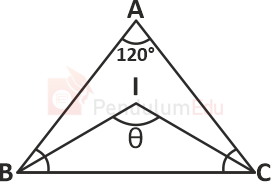Question of The Day28-02-2022

In a △ABC, the bisector of ∠B and ∠C meets at I, If ∠A = 120° what is the measure of ∠BIC?

Correct Answer : a ) 150°

Explanation :

According to the question

The bisector of ∠B and ∠C meets at I

∠A = 120°We know that

If the bisector of ∠B and ∠C of △ ABC meet at I, then ‘I’ will be the incenter of △ ABC

We know that

⇒∠I = 90° + ∠$$A \over 2$$

Substituting the values

⇒ ∠I=90+$$120 \over 2$$

⇒∠I = 150°

Hence, (a) is the correct answer.0